# Decibels

The sound intensity I may be expressed in decibels above the standard threshold of hearing I0. The expression is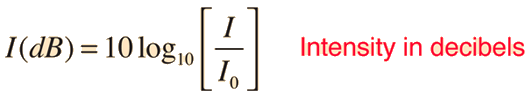The logarithm involved is just the power of ten of the sound intensity expressed as a multiple of the threshold of hearing intensity. Example: If I = 10,000 times the threshold, then the ratio of the intensity to the threshold intensity is 104, the power of ten is 4, and the intensity is 40 dB: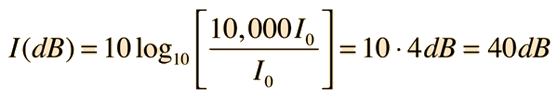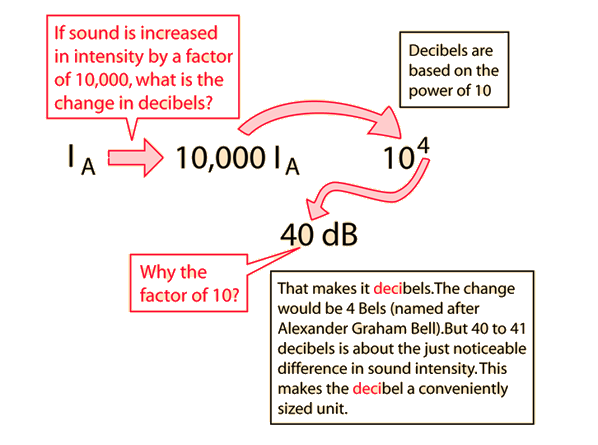The factor of 10 multiplying the logarithm makes it decibels instead of Bels, and is included because about 1 decibel is the just noticeable difference (JND) in sound intensity for the normal human ear.

Decibels provide a relative measure of sound intensity. The unit is based on powers of 10 to give a manageable range of numbers to encompass the wide range of the human hearing response, from the standard threshold of hearing at 1000 Hz to the threshold of pain at some ten trillion times that intensity.

Another consideration which prompts the use of powers of 10 for sound measurement is the rule of thumb for loudness: it takes about 10 times the intensity to sound twice as loud.

 Decibel calculation Dynamic levels of music in dB
 Power in dBm
Index

Sound level measurement

 HyperPhysics***** Sound R Nave
Go Back

# Decibels and Logarithms

The decibel scale is a reflection of the logarithmic response of the human ear to changes in sound intensity:The logarithm to the base 10 used in this expression is just the power of 10 of the quantity in brackets according to the basic definition of the logarithm: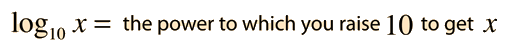Examples:Decibel calculation
Index

Sound level measurement

 HyperPhysics***** Sound R Nave
Go Back

# Decibel Calculation

The sound intensity in decibels above the standard threshold of hearing is calculated as a logarithm. If the intensity as a multiple of threshold is= x 10^then the intensity in decibels is given by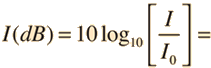dB

Decibels can also be used to express the relative intensity of two sounds. If one is expressed as a multiple of the other:

 IA = xIB = x 10^xIB

then the difference in decibels is given by

 IA = dB above IB

If you know the sound level in decibels at one distance in an open area, then you can estimate the dB level at another distance by making use of the inverse square law.

Index

Sound level measurement

 HyperPhysics***** Sound R Nave
Go Back

# JND in Sound Intensity

A useful general reference is that the just noticeable difference in sound intensity for the human ear is about 1 decibel.

JND = 1 decibel

In fact, the use of the factor of 10 in the definition of the decibel is to create a unit which is about the least detectable change in sound intensity.

That having been established, it can be noted that there are some variations. The jnd is about 1 dB for soft sounds around 30-40 dB at low and midrange freqencies. It may drop to 1/3 to 1/2 a decibel for loud sounds.

Caution must be used in applying the "one decibel" criterion. It presumes that you are increasing the same sound by one decibel. If you were adding a sound outside the critical band of frequency from this sound, you would be exciting fresh nerve endings, and the one decibel rule can't be presumed to apply. This causes some concern about the perceptual encoding schemes used with modern digital recording which might eliminate some significant audible content by the use of a "one decibel" criterion for dropping content.

 Illustration of variations
Index

Sound level measurement

 HyperPhysics***** Sound R Nave
Go Back

# Variations in Difference Threshold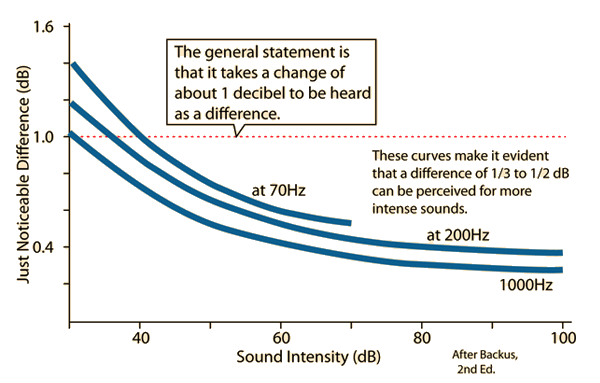The above data are from Backus, suggesting that the JND in dB is less for more intense sounds. He is citing Harvey Fletcher's "Speech and Hearing in Communication"(1953),p146, as the actual data source. But you can do a test for yourself of pairs of tones that are stated to be 2dB different at the McGraw-Hill site. This site discusses "Weber's law", which states just the opposite of the implication of the above curves.

 Just noticeable difference Decibels
Index

Sound level measurement

Reference
Backus

 HyperPhysics***** Sound R Nave
Go Back

# Power in dBm and Voltage in dBu

The sound intensity measured in the air is typically measured in decibels, partly because the logarithmic nature of decibels makes it possible to express a wide range of sound intensities with a small range of numbers. For the statement of the electric power associated with radio antennae, microwave sources and fiber optic sources, a wide range of numbers is encountered and it is convenient to use a logarithmic scale for that power. With a one milliwatt source as a standard power, the value 0 dBm is assigned to that standard. While that is an arbitrary choice of reference point, it turns out to be a practical reference level for radio applications. Once this standard is established, the relationship to other powers can be calculated in the same way that decibels of sound are handled. For example, 100 milliwatts dissipated in the load is 102 x the standard and is therefore 20 dBm.

For audio and radio applications, a standard application involved the dissipation of 1 milliwatt in a 600 ohm load. To get this power, corresponding to 0 dBm, a source voltage of 0.775 volts rms is required, and that voltage is often referred to as 0 dBu. This voltage can be obtained by using the AC power relationship for a 600 ohm resistive load in which the power dissipated is 1 milliwatt. For a resistive load the average power is Vrms2/R.

 Decibels Table of dBm values (Wiki) dBm tables
Index

Sound level measurement

Reference
Backus

 HyperPhysics***** Sound R Nave
Go Back